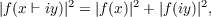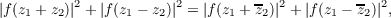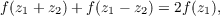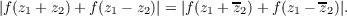#### Vol. 26, No. 1, 1968

 Download this articleFor screen For printingRecent Issues Vol. 325: 1 Vol. 324: 1  2 Vol. 323: 1  2 Vol. 322: 1  2 Vol. 321: 1  2 Vol. 320: 1  2 Vol. 319: 1  2 Vol. 318: 1  2Online Archive Volume: Issue:The Journal Subscriptions Editorial Board Officers Contacts Submission Guidelines Submission Form Policies for Authors ISSN: 1945-5844 (e-only) ISSN: 0030-8730 (print) Special Issues Author Index To Appear Other MSP Journals
On inequalities generalizing a Pythagorean functional equation and Jensen’s functional equation

### Hiroshi Haruki

Vol. 26 (1968), No. 1, 85–90
##### Abstract

Several authors have solved the Pythagorean functional equation(1)

where f is an entire function and x and y are real variables.

A simple computation shows that, if f is a solution of (1), then f is also a solution of(2)

where z1 and z2 are complex variables. (If an entire function vanishes at the origin and is a solution of (2), then it is a solution of (1), and conversely.) If an entire function f is a solution of Jensen’s functional equation(3)

where z1 and z2 are complex variables, then it is also a solution of(4)

In this paper we shall prove that a solution of (4) is always a solution of (2). Then we shall solve certain functional inequalities derived from (2) and use the solutions to solve (1), (2), (3), and (4).

Primary: 39.30
##### Milestones